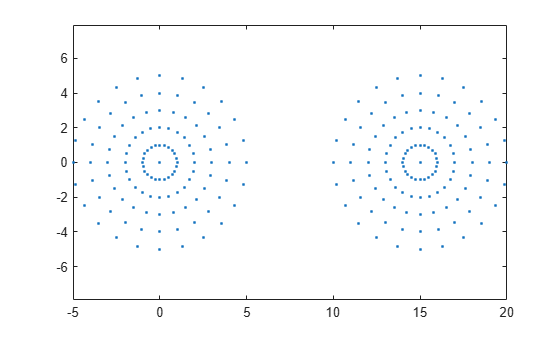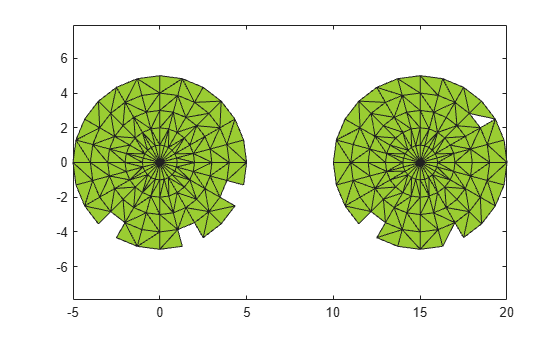# criticalAlpha

Alpha radius defining critical transition in shape

## Syntax

``a = criticalAlpha(shp,type)``

## Description

example

````a = criticalAlpha(shp,type)` returns the critical alpha radius that produces a notable transition in the alpha shape. Specifying `type` as `'all-points'` returns the smallest alpha radius producing an alpha shape that encloses all points. Specifying `type` as `'one-region'` returns the smallest alpha radius producing an alpha shape that encloses all points and has only one region.```

## Examples

collapse all

Create and plot a set of 2-D points.

```th = (pi/12:pi/12:2*pi)'; x1 = [reshape(cos(th)*(1:5), numel(cos(th)*(1:5)),1); 0]; y1 = [reshape(sin(th)*(1:5), numel(sin(th)*(1:5)),1); 0]; x = [x1; x1+15;]; y = [y1; y1]; plot(x,y,'.') axis equal```Create an alpha shape using the default alpha radius.

`shp = alphaShape(x,y);`

Compute the smallest alpha radius that produces an alpha shape enclosing all of the points and plot the corresponding alpha shape.

```pc = criticalAlpha(shp,'all-points'); shp.Alpha = pc; plot(shp)```Compute the smallest alpha radius that produces an alpha shape enclosing all of the points and having only one region.

```shp = alphaShape(x,y); pc = criticalAlpha(shp,'one-region'); shp.Alpha = pc; plot(shp)```## Input Arguments

collapse all

Alpha shape, specified as an `alphaShape` object. For more information, see `alphaShape`.

Example: `shp = alphaShape(x,y)` creates a 2-D `alphaShape` object from the `(x,y)` point coordinates.

Type of critical transition, specified as either `'all-points'` or `'one-region'`.

• `'all-points'` corresponds to the smallest alpha radius producing an alpha shape that encloses all points.

• `'one-region'` corresponds to the smallest alpha radius producing an alpha shape that encloses all points and has only one region.

Data Types: `char`

## Output Arguments

collapse all

Critical alpha radius, returned as a scalar. `a` is the value of the alpha radius that produces an alpha shape, which either encloses all points (if `type` is `'all-points'`), or encloses all points within a single region (if `type` is `'one-region'`).

After using `criticalAlpha` to find `a`, you can make the alpha radius of `shp` equal to `a` by typing `shp.Alpha = a`.

## Version History

Introduced in R2014b